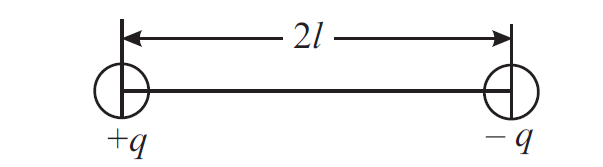# Dipole & Dipole Moment – definition, formula

Last updated on March 24th, 2022 at 04:06 pm

In this post, we will find out what exactly an electric dipole is. We will also define dipole moment and find out the formula of dipole moment.

## Dipole | Electric Dipole

If two equal and opposite charges are separated by a small distance, the system is said to form a dipole. The most familiar example is H2O.

Figure 1 shows charges +q and – q separated by a small distance 2l.Figure 1: Two unlike charges of equal magnitude separated by a small distance form a dipole.

## Dipole Moment

The product of the magnitude of charge and separation between the charges in a dipole is called dipole moment, p.

To get the magnitude of the charge of a dipole take the magnitude of any member charge, as magnitude wise both charges are the same.

The equation of the dipole moment p is as follows: p = q × 2l ………………. (1)

Its SI unit is coulomb-metre.

The dipole moment is a vector quantity. Equation (1) gives its magnitude and its direction is from negative charge to positive charge along the line joining the two charges (axis of the dipole).

The magnitude of the dipole moment: p = q × 2l

The direction of the dipole moment: from negative charge to positive charge along the line joining the two charges (axis of the dipole)

The net charge on an electric dipole is zero.

Having defined a dipole and dipole moment, we are now in a position to calculate the electric field due to a dipole. Read this post: electric field due to a dipole on its axis and on its equatorial line – with formulas

Also read: Torque on an electric dipole